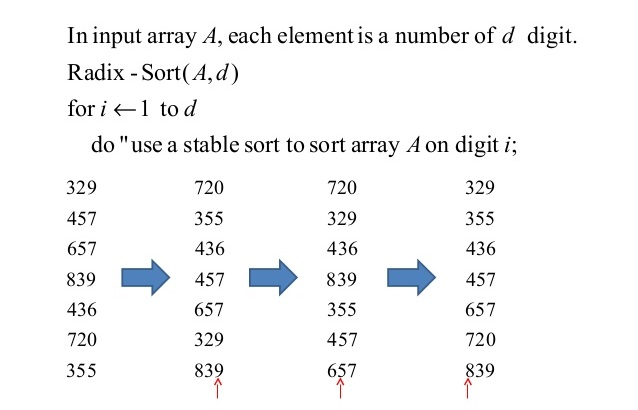suggest change

Radix Sort is lower bound comparison based algorithm. It is a non-comparative integer sorting algorithm that sorts data with integer keys by grouping keys by individual digits which share some significant position and value. Radix sort is a linear time sorting algorithm that sort in O(n+k) time when elements are in range from 1 to k. The idea of Radix Sort is to do digit by digit sort starting from least significant digit to most significant digit. Radix sort uses counting sort as a subroutine to sort. Radix sort is generalization of bucket sort.

Pseudo code for Bucket Sort:

1. Create an array of [0..n-1] elements.
2. Call Bucket Sort repeatedly on least to most significant digit of each element as the key.
3. Return the sorted array.Space Auxiliary: `O(n)`

Time Complexity: `O(n)`